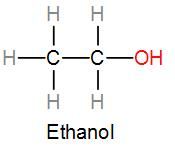QuestionAnswers

# Which bond is not present in ethanol $C{{H}_{3}}C{{H}_{2}}OH$?[A] C-C[B] H-H[C] H-O[D] O-CVerified
129.6k+ views
Hint:
Ethanol is an alcohol which means it will contain a –OH functional group and therefore it has a carbon-oxygen bond. To find out which of the given bonds is not present in ethanol, we can draw its structure and the formula is given which is $C{{H}_{3}}C{{H}_{2}}OH$.

Ethanol is a chemical compound with the chemical formula ${{C}_{2}}{{H}_{5}}OH$ we can also write is as $C{{H}_{3}}C{{H}_{2}}OH$.
As we can see, ethanol contains a –OH functional group, therefore it is termed as alcohol.
We also know ethanol as ethyl alcohol because it contains an ethyl group which is $C{{H}_{3}}C{{H}_{2}}$ but we can also write it as ${{C}_{2}}{{H}_{5}}$ and the alcohol functional group, giving it the name ethyl alcohol.
Ethanol is a simple alcohol and we can naturally produce it by fermentation of sugar by yeast but also synthetically through petrochemical processes.
We commonly use ethanol as a chemical solvent to dissolve compounds which are insoluble in non-polar solvents.
If we draw the structure of ethanol showing the connectivity of the bonds, we can answer which bond is not present in ethanol. Structure of ethanol can be drawn as-As we can see from the above diagram, ethanol has carbon-hydrogen bonds, carbon-carbon bond and also a carbon-hydrogen bond. Therefore, the only bond not present in ethanol among the given options is a hydrogen-hydrogen bond.

Therefore, the correct answer is option [B] H-H, Hydrogen-Hydrogen bond.

Note:
We should not confuse ethanol with methanol or even ethanal. Methanol is also an alcohol but it contains a methyl group and its formula is $C{{H}_{3}}OH$ and ethanal is a completely different chemical compound. Ethanal is not even an alcohol, it is an aldehyde and it is commonly known as acetaldehyde. Formula of ethanal is $C{{H}_{3}}CHO$.